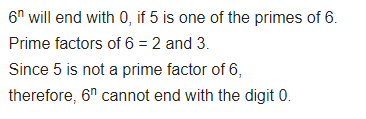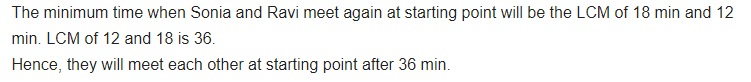• +91 9971497814
• info@interviewmaterial.com

# Real Numbers Ex-1.2 Interview Questions Answers

### Related Subjects

Question 1 : Express each number as a product of its prime factors:-

(i) 140

(ii) 156

(iii) 3825

(iv) 5005

(v) 7429

Solutions:

(i) 140

We will get the product of its prime factor.

140 = 2× 2 × 5 × 7 × 1 = 22×5×7

Q ii      156

Solutions:

(ii)156

We will get the product of its prime factor.

156 = 2× 2 × 13 × 3 × 1 = 22× 13 × 3

Q iii     3825

Solutions:

(iii) 3825

We will get the product of its prime factor.

3825 =3 × 3 × 5 × 5 × 17 × 1 = 32×52×17

Q iv     5005

Solutions:

(iv) 5005

We will get the product of its prime factor.

5005 = 5 × 7 × 11 × 13 × 1 = 5 × 7 × 11 × 13

Q v      7429

Solutions:

(v)7429

We will get the product of its prime factor.

7429 =17 × 19 × 23 × 1 = 17 × 19 × 23

Question 2 : Find the LCM and HCF of the following pairs of integers and verify thatLCM × HCF = product of the two numbers.

(i) 26 and 91

(ii) 510 and 92

(iii) 336 and 54

(i) 26 and 91

Solutions:

We will get the product of its prime factor

26 = 2 × 13 × 1

91 = 7 × 13 × 1

LCM(26, 91) = 2 × 7 × 13 × 1 = 182

And HCF (26, 91) = 13

Verification

Now, product of 26 and 91 = 26 × 91 = 2366

And Product of LCM and HCF = 182 × 13 = 2366

Hence, LCM × HCF = product of the 26 and 91.

(ii) 510 and 92

Solutions:

We will get the product of its prime factor

510 = 2 × 3 × 17 × 5 × 1

92 = 2 × 2 × 23 × 1

LCM(510, 92) = 2 × 2 × 3 × 5 × 17 × 23 = 23460

And HCF (510, 92) = 2

Verification

Now, product of 510 and 92 = 510 × 92 = 46920

And Product of LCM and HCF = 23460 × 2 = 46920

Hence, LCM × HCF = product of the 510 and 92.

(iii) 336 and 54

Solutions:

We will get the product of its prime factor

336 = 2 × 2 × 2 × 2 × 7 × 3 × 1

54 = 2 × 3 × 3 × 3 × 1

LCM(336, 54) = = 3024

And HCF(336, 54) = 2×3 = 6

Verification

Now, product of 336 and 54 = 336 × 54 = 18,144

And Product of LCM and HCF = 3024 × 6 = 18,144

Hence, LCM × HCF = product of the 336 and 54.

Question 3 :

Find the LCM and HCF of the following integersby applying the prime factorisation method.

(i) 12, 15 and 21

(ii) 17, 23 and 29

(iii) 8, 9 and 25

Q (i)     12,15 and 21

Solutions:

(i) 12, 15 and 21

We will get the product of its prime factor

12=2×2×3

15=5×3

21=7×3

Therefore,

HCF(12,15,21) = 3

LCM(12,15,21) = 2 × 2 × 3 × 5 × 7 = 420

Q (ii)    17,23 and 29

(ii)17, 23 and 29

We will get the product of its prime factor

17=17×1

23=23×1

29=29×1

Therefore,

HCF(17,23,29) = 1

LCM(17,23,29) = 17 × 23 × 29 = 11339

Q (iii)   8, 9 and 25

(iii)8, 9 and 25

We will get the product of its prime factor

8=2×2×2×1

9=3×3×1

25=5×5×1

Therefore,

HCF(8,9,25)=1

LCM(8,9,25) = 2×2×2×3×3×5×5 = 1800

Question 4 :

Given that HCF (306, 657) = 9, find LCM (306,657).

Solution:

We know that,

HCF×LCM=Product of the two given numbers

Then,

9 × LCM = 306 × 657

LCM = (306×657)/9 = 22338

Hence, LCM(306,657) = 22338

Question 5 : Check whether 6n can end with the digit 0 for anynatural number n.

Answer 5 :Question 6 : Explain why 7 × 11 × 13 + 13 and 7 × 6 × 5 × 4 × 3 × 2 × 1 + 5 arecomposite numbers.

We know, if a number is composite, then it means it has factorsother than 1 and itself. Therefore,

Given

7 × 11 × 13 + 13

Taking 13 as common factor

We get,

=13(7×11×1+1) = 13(77+1) = 13×78 = 13×3×2×13

Hence, 7 × 11 × 13 + 13 is a composite number.

Now let’s take the other number,

7 × 6 × 5 × 4 × 3 × 2 × 1 + 5

Taking 5 as a common factor, we get,

=5(7×6×4×3×2×1+1) = 5(1008+1) = 5×1009

Hence, 7 × 6 × 5 × 4 × 3 × 2 × 1 + 5 is acomposite number.

Question 7 :

There is a circular path around a sportsfield. Sonia takes 18 minutes to drive one round of the field, while Ravi takes12 minutes for the same. Suppose they both start at the same point and at thesame time, and go in the same direction. After how many minutes will they meetagain at the starting point?

Answer 7 :Todays Deals### Real Numbers Ex-1.2 Contributorskrishan

Name:
Email:

# Latest News# 9000 interview questions in different categories